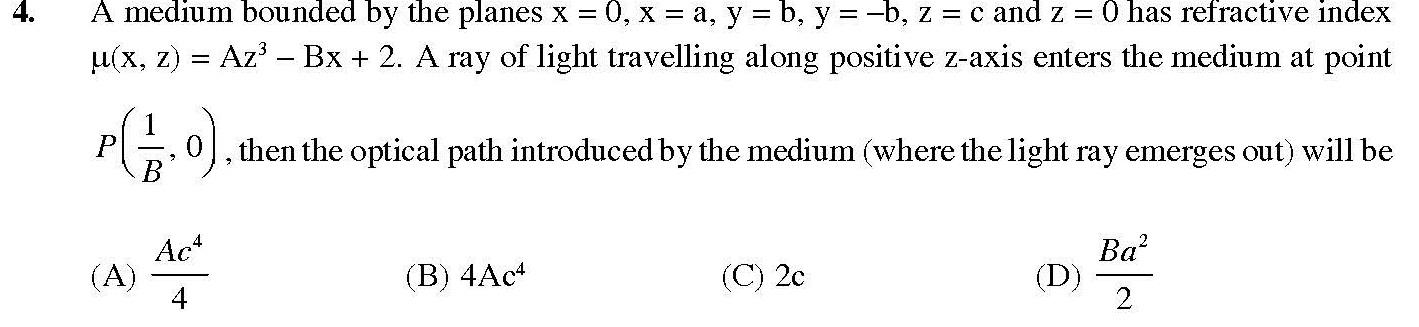# Refraction•## 1 Answers

Aditya Agarwal ·

The rays are travelling perp. to the plane having different optical media.
So the rays wont get deflected.

Since the rays enter at x = 1/B,
the U function becomes a function of z only.

u(z) = Az4+1

Total Optical path the ray traverses = 0c∫udz = Ac44+c
Optical path introduced = Ac44+c - c = Ac44